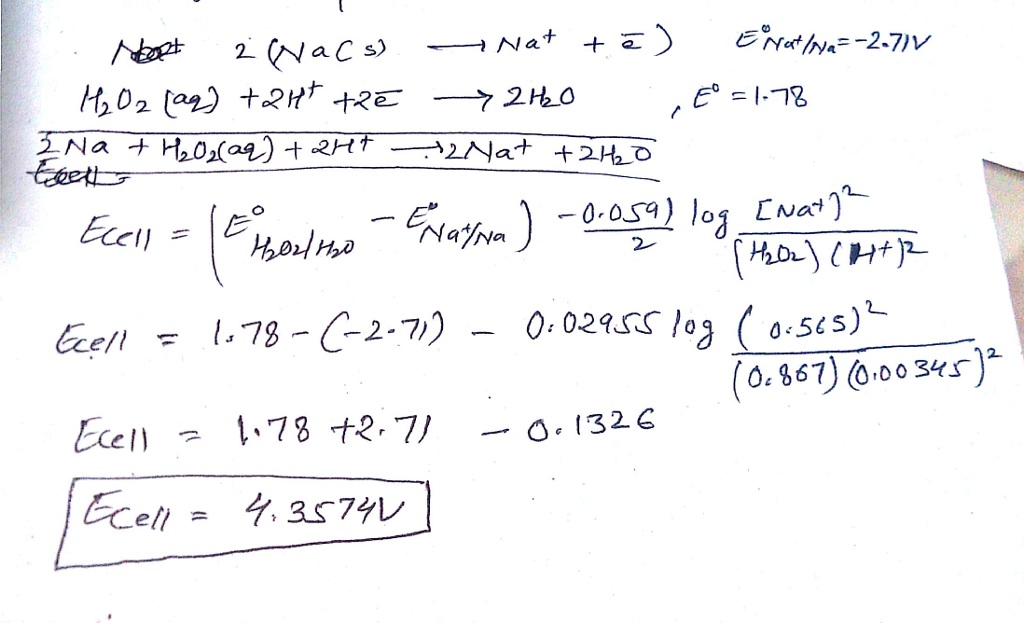# Question & Answer: A galvanic cell is based on the following half-reactions at 285 K: Na+ + e- → Na Eo = -2.71 V…..

A galvanic cell is based on the following half-reactions at 285 K: Na+ + e- → Na Eo = -2.71 V H2O2 (aq) + 2 H+ + 2 e- → 2 H2O Eo = 1.78 V What will the potential of this cell be when [Na+] = 0.565 M, [H+] = 0.00345 M, and [H2O2] = 0.867 M?